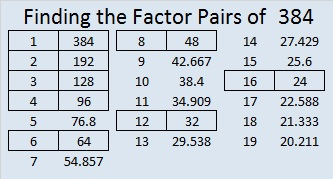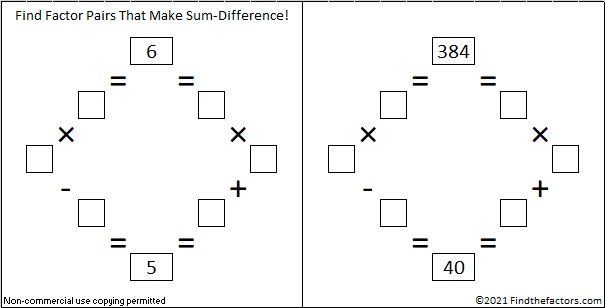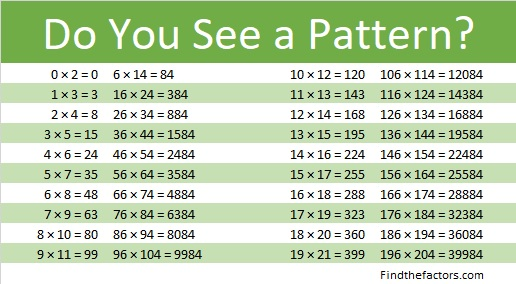# 384 and Level 6

Contents

### Today’s Puzzle:Print the puzzles or type the factors on this excel file: 10 Factors 2015-02-02

Here is a logical way to use the clues in the puzzle to find the solution:### Factors of 384:

384 is even and, therefore, composite.

• 384 is a composite number.
• Prime factorization: 384 = 2 x 2 x 2 x 2 x 2 x 2 x 2 x 3, which can be written 384 = (2^7) x 3
• The exponents in the prime factorization are 7 and 1. Adding one to each and multiplying we get (7 + 1)(1 + 1) = 8 x 2 = 16. Therefore 384 has exactly 16 factors.
• Factors of 384: 1, 2, 3, 4, 6, 8, 12, 16, 24, 32, 48, 64, 96, 128, 192, 384
• Factor pairs: 384 = 1 x 384, 2 x 192, 3 x 128, 4 x 96, 6 x 64, 8 x 48, 12 x 32, or 16 x 24
• Taking the factor pair with the largest square number factor, we get √384 = (√6)(√64) = 8√6 ≈ 18.596### Sum-Difference Puzzles:

6 has two factor pairs. One of those pairs adds up to 5, and the other one subtracts to 5. Put the factors in the appropriate boxes in the first puzzle.

384 has eight factor pairs. One of the factor pairs adds up to 40, and a different one subtracts to 40. If you can identify those factor pairs, then you can solve the second puzzle!The second puzzle is really just the first puzzle in disguise. Why would I say that?

### More about the Number 384:

384 is in this cool pattern:This site uses Akismet to reduce spam. Learn how your comment data is processed.